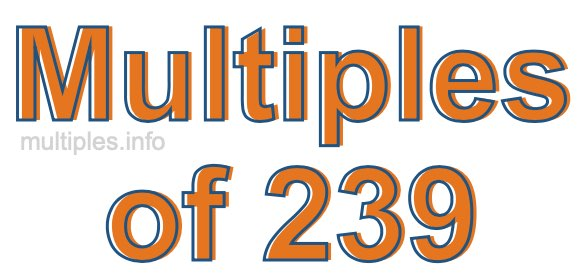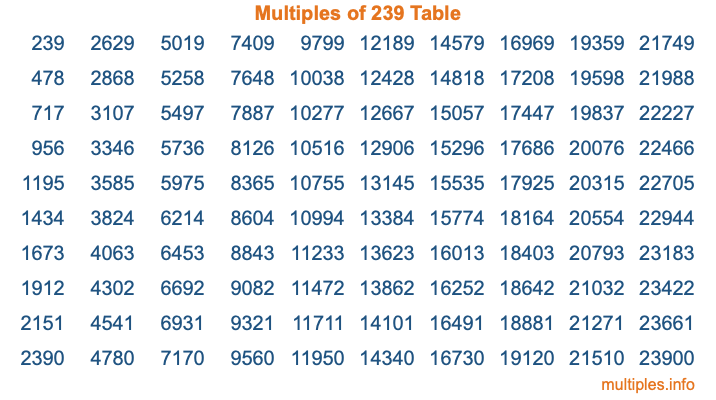Multiples of 239Welcome to the Multiples of 239 page. Here we will first teach you everything you will ever need to know about the multiples of 239, and then give you a study guide summary of everything we taught you to make sure you remember it all. Use this page to look up facts and learn information about the multiples of 239. This page will make you a multiples of two hundred thirty-nine expert!

Definition of Multiples of 239
Multiples of 239 are all the numbers that when divided by 239 equal an integer. Each of the multiples of 239 are called a multiple. A multiple of 239 is created by multiplying 239 by an integer.

Therefore, to create a list of multiples of 239, you start with 1 multiplied by 239, then 2 multiplied by 239, then 3 multiplied by 239, and so on for as long as you want. Thus, the list of the first five multiples of 239 is 239, 478, 717, 956, and 1195. To see a larger list of multiples of 239, see the printable image of Multiples of 239 further down on this page. We also have a category where you can choose any nth multiple of 239.

Multiples of 239 Checker
The Multiples of 239 Checker below checks to see if any number of your choice is a multiple of 239. In other words, it checks to see if there is any number (integer) that when multiplied by 239 will equal your number. To do that, we divide your number by 239. If the the quotient is an integer, then your number is a multiple of 239.

Is  a multiple of 239?

Least Common Multiple of 239 and ...
A Least Common Multiple (LCM) is the lowest multiple that two or more numbers have in common. This is also called the smallest common multiple or lowest common multiple and is useful to know when you are adding our subtracting fractions. Enter one or more numbers below (239 is already entered) to find the LCM.

Check out our LCM Calculator if you need more details about the Least Common Multiple or if you need the LCM for different numbers for adding and subtraction fractions.

nth Multiple of 239
As we stated above, 239 is the first multiple of 239, 478 is the second multiple of 239, 717 is the third multiple of 239, and so on. Enter a number below to find the nth multiple of 239.

th multiple of 239

Multiples of 239 vs Factors of 239
239 is a multiple of 239 and a factor of 239, but that is where the similarities end. All postive multiples of 239 are 239 or greater than 239. All positive factors of 239 are 239 or less than 239.

Below is the beginning list of multiples of 239 and the factors of 239 so you can compare:

Multiples of 239: 239, 478, 717, 956, 1195, etc.

Factors of 239: 1, 239

As you can see, the multiples of 239 are all the numbers that you can divide by 239 to get a whole number. The factors of 239, on the other hand, are all the whole numbers that you can multiply by another whole number to get 239.

It's also interesting to note that if a number (x) is a factor of 239, then 239 will also be a multiple of that number (x).

Multiples of 239 vs Divisors of 239
The divisors of 239 are all the integers that 239 can be divided by evenly. Below is a list of the divisors of 239.

Divisors of 239: 1, 239

The interesting thing to note here is that if you take any multiple of 239 and divide it by a divisor of 239, you will see that the quotient is an integer.

Multiples of 239 Table
Below is an image of the first 100 multiples of 239 in a table. The table is in chronological order, column by column. The first column has the first ten multiples of 239, the second column has the next ten multiples of 239, and so on.The Multiples of 239 Table is also referred to as the 239 Times Table or Times Table of 239. You are welcome to print out our table for your studies.

Negative Multiples of 239
Although not often discussed or needed in math, it is worth mentioning that you can make a list of negative multiples of 239 by multiplying 239 by -1, then by -2, then by -3, and so on, to get the following list of negative multiples of 239:

-239, -478, -717, -956, -1195, etc.

Multiples of 239 Summary
Below is a summary of important Multiples of 239 facts that we have discussed on this page. To retain the knowledge on this page, we recommend that you read through the summary and explain to yourself or a study partner why they hold true.

There are an infinite number of multiples of 239.

A multiple of 239 divided by 239 will equal a whole number.

239 divided by a factor of 239 equals a divisor of 239.

The nth multiple of 239 is n times 239.

The largest factor of 239 is equal to the first positive multiple of 239.

239 is a multiple of every factor of 239.

239 is a multiple of 239.

A multiple of 239 divided by a divisor of 239 equals an integer.

239 divided by a divisor of 239 equals a factor of 239.

Any integer times 239 will equal a multiple of 239.

Multiples of a Number
Here you can get the multiples of another number, all with the same attention to detail as we did for multiples of 239 on this page.

Multiples of
Multiples of 240
Did you find our page about multiples of two hundred thirty-nine educational? Do you want more knowledge? Check out the multiples of the next number on our list!